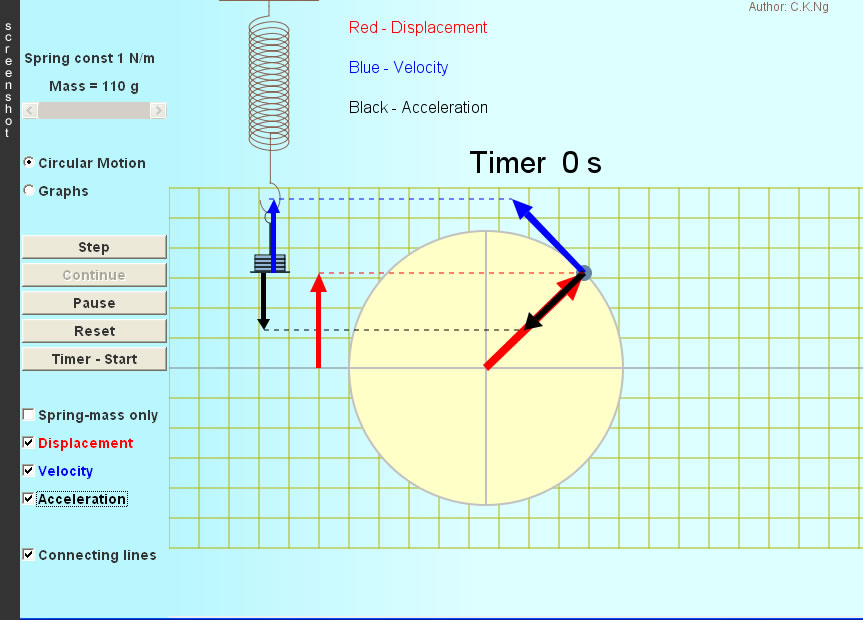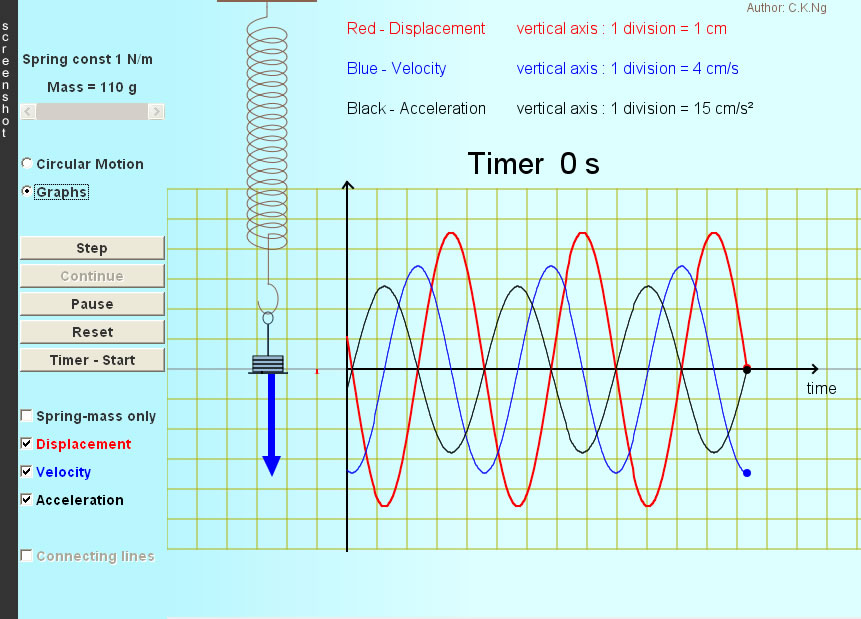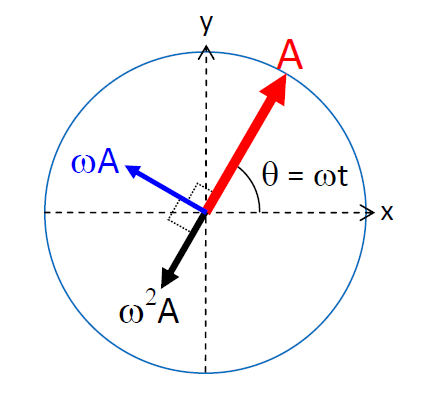Spring-Mass SHM (Kinematics)

To begin an oscillation, drag the block up or down and then release.

The periodic motion of the block is simple harmonic because the acceleration is always proportional, but opposite to the displacement from the equilibrium position (definition of SHM).

Properties of SHM:

1. It is identical to the projection of a uniform circular motion on an axis.
2. The curves (x-t, v-t and a-t) are sinusoidal with acceleration leading velocity by π/2 and velocity leading displacement by π/2.
3. The period of oscillation is independent of amplitude (isochronism).

 Internal Links : Spring-Mass SHM (Energy) Pendulum SHM Oscillator Phase DifferenceSuppose a particle P rotating anticlockwise with radius A and angular frequency ω. Of P, the displacement from the center of circle, the tangential velocity (ωA) and the centripetal acceleration (ω2A) are represented, respectively, by the red, blue, and black vectors in the above figures. Suppose the displacement vector makes angle θ = ωt with the positive x-axis, so the projection of this vector on the y-axis is y = Asinθ = A sin(ωt) ....(1) The tangential velocity leads the displacement by π/2, so the projection of the velocity vector on the y-axis is vy =ωA sin(ωt + π/2) ....(2) The centripetal acceleration leads the displacement by π, so the projection of the acceleration vector on the y-axis is ay =ω2Asin(ωt +π) ....(3) Using (1), (2) and the identity sin(θ + π) = -sinθ , we obtain ay = -ω2y, the SHM equation. Thus, we conclude A SHM can be regarded as an axis-projection of a uniform circular motion. In shm, the amplitudes of displacement, velocity and acceleration are A, ωA and ω2A respectively. Phase Differences: a leads v by π/2 ; v leads displacement by π/2.

 The author (Chiu-king Ng) has the copyright on all the simulations in this website. Email phyAA@phy.hk, where AA is the prime number following 7. Last Update:2019-3-20